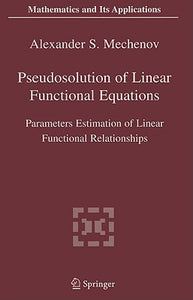# Pseudosolution Of Linear Functional Equations: Parameters Estimation Of Linear Functional Relationships (mathematics And Its Applications)

Free shipping (Guaranteed delivery 3-5 business days for In-Stock products)
Out of stock

## ISBN details

• ISBN 10: 0387245057
• ISBN 13: 9780387245058

## Overview

In the book there are introduced models and methods of construction of pseudo-solutions for the well-posed and ill-posed linear functional equations circumscribing models passive, active and complicated experiments. Two types of the functional equations are considered: systems of the linear algebraic equations and linear integral equations. Methods of construction of pseudos6lutions are developed in the presence of passive right-hand side errors for two types of operator errors: passive measurements and active representation errors of the operator, and all their combinations. For the determined and stochastic models of passive experiments the method of the least distances of construction of pseudosolutions is created, the maximum likelihood method of construction of pseudosolutions is applied for active experiments, and then methods for combinations of models of regression, of passive and of active experiments are created. We have constructed regularized variants of these methods for systems of the linear algebraic equations with the degenerated matrices and for linear integral equations of the first kind. In pure mathematics, the solution techniques of the functional equations with exact input data more often are studied. In applied mathematics, problem consists in construction of pseudosolutions, that is, solution of the hctional equations with perturbed input data. Such problem in many cases is incomparably more complicated. The book is devoted to a problem of construction of a pseudosolution (the problem of a parameter estimation) in the following fundamental sections of applied mathematics: confluent models passive, active and the every possible mixed experiments.

## Other Details

• Publisher: Springer
• Edition: 2005
• Dimensions: 9.21 (w) x 6.14 (h) x 0.63 (d)
• Pages: 238
• Date Published: 2005
• Authors: Alexander S. Mechenov